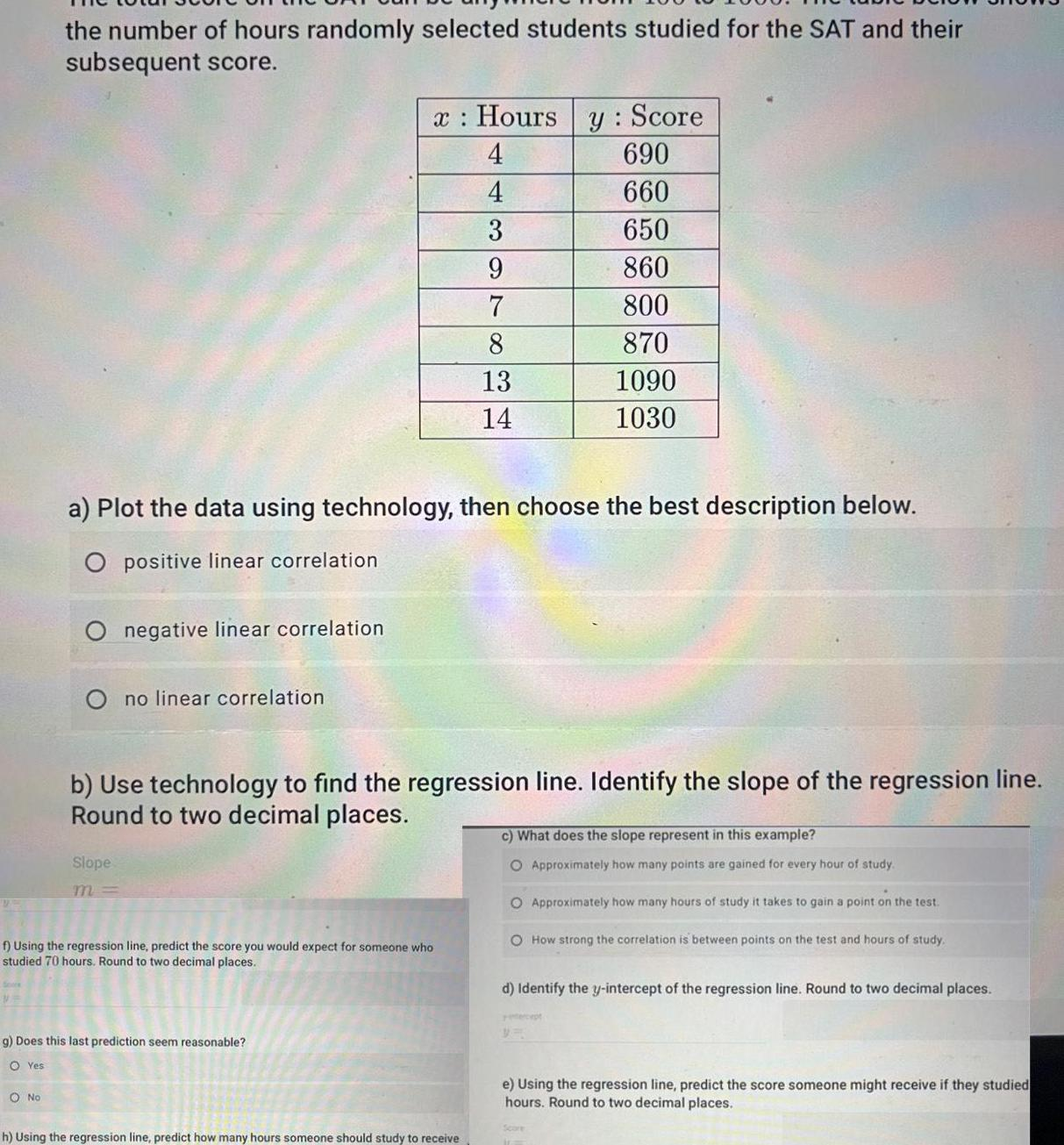Question:

# the number of hours randomly selected students studied for

Last updated: 9/18/2023the number of hours randomly selected students studied for the SAT and their subsequent score No a Plot the data using technology then choose the best description below O positive linear correlation negative linear correlation Slope m no linear correlation x Hours y Score 4 690 4 660 3 650 9 860 7 800 8 870 13 1090 14 1030 b Use technology to find the regression line Identify the slope of the regression line Round to two decimal places f Using the regression line predict the score you would expect for someone who studied 70 hours Round to two decimal places g Does this last prediction seem reasonable OYes h Using the regression line predict how many hours someone should study to receive c What does the slope represent in this example O Approximately how many points are gained for every hour of study O Approximately how many hours of study it takes to gain a point on the test O How strong the correlation is between points on the test and hours of study d Identify the y intercept of the regression line Round to two decimal places e Using the regression line predict the score someone might receive if they studied hours Round to two decimal places